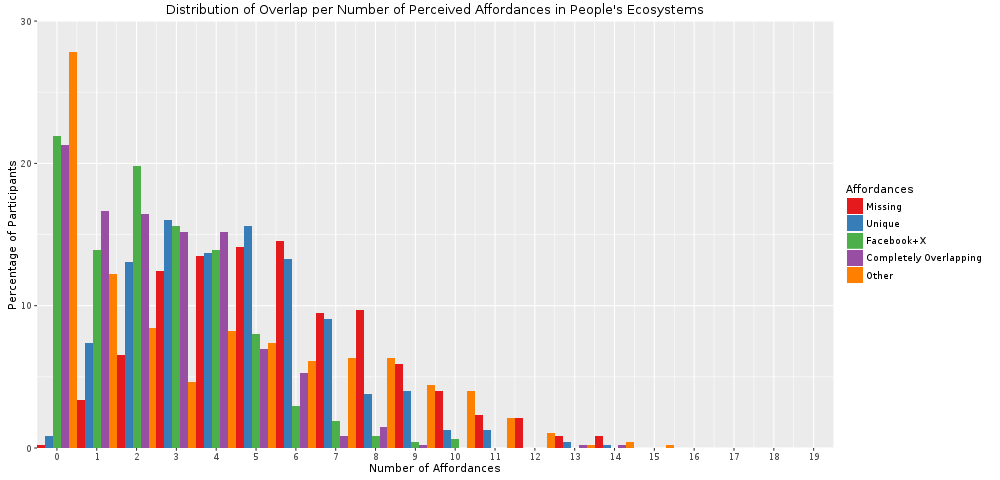import pandas as pd
import numpy as np
import matplotlib.pyplot as plt
import csv

%load_ext rpy2.ipython
aff_distribution = pd.DataFrame.from_csv('./aff_distribution_data.csv',sep=',', index_col=False)
%Rpush aff_distribution
%R install.packages('reshape')
%R library('reshape')
%R library('ggplot2')

The rpy2.ipython extension is already loaded. To reload it, use:

/srv/paws/lib/python3.4/site-packages/rpy2/robjects/functions.py:106: UserWarning: Installing package into ‘/usr/local/lib/R/site-library’
(as ‘lib’ is unspecified)

res = super(Function, self).__call__(*new_args, **new_kwargs)
/srv/paws/lib/python3.4/site-packages/rpy2/robjects/functions.py:106: UserWarning: trying URL 'https://cloud.r-project.org/src/contrib/reshape_0.8.5.tar.gz'

res = super(Function, self).__call__(*new_args, **new_kwargs)
/srv/paws/lib/python3.4/site-packages/rpy2/robjects/functions.py:106: UserWarning: Content type 'application/x-gzip'
res = super(Function, self).__call__(*new_args, **new_kwargs)
/srv/paws/lib/python3.4/site-packages/rpy2/robjects/functions.py:106: UserWarning:  length 42350 bytes (41 KB)

res = super(Function, self).__call__(*new_args, **new_kwargs)
/srv/paws/lib/python3.4/site-packages/rpy2/robjects/functions.py:106: UserWarning: =
res = super(Function, self).__call__(*new_args, **new_kwargs)
/srv/paws/lib/python3.4/site-packages/rpy2/robjects/functions.py:106: UserWarning:

res = super(Function, self).__call__(*new_args, **new_kwargs)

res = super(Function, self).__call__(*new_args, **new_kwargs)

The downloaded source packages are in

array(['reshape', 'ggplot2', 'tools', 'stats', 'graphics', 'grDevices',
'utils', 'datasets', 'methods', 'base'],
dtype='<U9')
%%R -w 1000
aff_distribution
cbPalette <- c("#e41a1c", "#377eb8","#4daf4a", "#984ea3", "#ff7f00")
mdata <- melt(aff_distribution, id=c("Number.of.Affordances"))

plt = ggplot(mdata, aes(y=value, x=Number.of.Affordances,fill=variable,width=1)) + geom_bar(position="dodge",stat="identity")
plt = plt+scale_fill_manual(name="Affordances",labels=c("Missing", "Unique", "Facebook+X", "Completely Overlapping", "Other"),values=cbPalette)+scale_x_continuous(breaks=0:19,expand=c(0,0))
plt = plt + scale_y_continuous(limits=c(0,30),expand=c(0,0))
plt = plt + labs(title = "Distribution of Overlap per Number of Perceived Affordances in People's Ecosystems",
x="Number of Affordances", y="Percentage of Participants")
plt